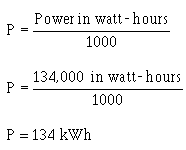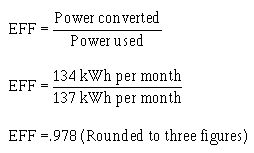Power conversion and efficiencyCustom SearchPower ratingMatter, Energy, and ElectricitySeries DC circuitsPOWER CONVERSION AND EFFICIENCY

The term power consumption is common in the electrical field. It is applied to the use of power in the same sense that gasoline consumption is applied to the use of fuel in an automobile.

Another common term is power conversion.
Power is used by electrical devices and is converted from one form of energy to another. An electrical motor converts electrical energy to mechanical energy. An electric light bulb converts electrical energy into light energy and an electric range converts electrical energy into heat energy. Power used by electrical devices is measured in energy. This practical unit of electrical energy is equal to 1 watt of power used continuously for 1 hour. The term kilowatt hour (kWh) is used more extensively on a daily basis and is equal to 1,000 watt-hours.

The EFFICIENCY of an electrical device is the ratio of power converted to useful energy divided by the power consumed by the device. This number will always be less than one (1.00) because of the losses in any electrical device. If a device has an efficiency rating of .95, it effectively transforms 95 watts into useful energy for every 100 watts of input power. The other 5 watts are lost to heat, or other losses which cannot be used.

Calculating the amount of power converted by an electrical device is a simple matter. You need to know the length of time the device is operated and the input power or horsepower rating. Horsepower, a unit of work, is often found as a rating on electrical motors. One horsepower is equal to 746 watts. Example: A 3/4-hp motor operates 8 hours a day. How much power is converted by the motor per month? How many kWh does this represent?

Given:

t = 8 hrs x 30 days

P = 3/4 hp

Solution: Convert horsepower to watts

P = hp x 746 watts

P = 3/4 x 746 watts

P = 559 watts

Convert watts to watt-hours

P = work x time

P = 559 watts x 8 x 30

P = 134,000 watt-hours per month

(NOTE: These figures are rounded to the nearest 1000.)

To convert to kWhIf the motor actually uses 137 kWh per month, what is the efficiency of the motor?

Given:

Power converted = 134 kWh per month

Power used = 137 kWh per month

Solution:How much power is converted by a 1-horsepower motor in 12 hours?

What is the efficiency of the motor if it actually uses 9.5 kWh in 12 hours?Integrated Publishing, Inc. - A (SDVOSB) Service Disabled Veteran Owned Small Business